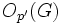# P-constraint is not quotient-closed

This article gives the statement, and possibly proof, of a group property (i.e., p-constrained group) not satisfying a group metaproperty (i.e., quotient-closed group property).
View all group metaproperty dissatisfactions | View all group metaproperty satisfactions|Get help on looking up metaproperty (dis)satisfactions for group properties
Get more facts about p-constrained group|Get more facts about quotient-closed group property|

## Statement

We can have a finite group$G$, a prime number$p$, and a normal subgroup$N$ of$G$ such that$G$ is a p-constrained group but$G/N$ is not a p-constrained group.

## Facts used

1. Constrained for a prime divisor implies not simple non-abelian

## Proof

Let$G$ be the wreath product of Z2 and A5 defined as the wreath product with base group cyclic group:Z2 and acting group alternating group:A5, where we use the natural permutation action of the acting group on a set of five elements. More explicitly,$G$ is the external semidirect product of elementary abelian group:E32 and alternating group:A5 where the latter acts on the former by coordinate permutations induced by the permutations on a set of five elements.

The group$G$ has order$\! 2^5 \cdot 60 = 1920 = 2^7 \cdot 3 \cdot 5$

Let$p = 2$.

We note that:

1.$G$ is$p$-constrained: Indeed,$O_{p'}(G)$ is trivial, and$O_{p',p}(G)$ is the base of the semidirect product, i.e., a normal subgroup isomorphic to elementary abelian group:E32. In particular, this is contained in any$p$-Sylow subgroup$P$, so$P \cap O_{p',p}(G)$ is also the normal subgroup that forms the base of the semidirect product. The subgroup is a self-centralizing normal subgroup, because it is an abelian normal subgroup and the induced action by the quotient is faithful. Thus, we get the condition$C_G(P \cap O_{p',p}(G)) \le O_{p,p}(G)$.
2.$G/O_{p',p}(G)$ is not$p$-constrained: Since$O_{p',p}(G)$ is the normal subgroup that forms the base of the semidirect product, the quotient group is alternating group:A5, which is a simple non-abelian group, with$p$ dividing its order, hence by Fact (1) is not$p$-constrained.